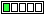All about flooble | fun stuff | Get a free chatterbox | Free JavaScript | Avatarsperplexus dot infoDon't be confused (Posted on 2016-11-14)If x satisfy the equation x^4 - x^2 + 1 = 0 , find the value of x^5 + 1/x

 No Solution Yet Submitted by Danish Ahmed Khan No RatingComments: ( Back to comment list | You must be logged in to post comments.)using a calculator| Comment 3 of 4 |Using the quadratic formula to solve for x^2:

x^2 = (1 +/- sqrt(1-4))/2

x^2 = (1 +/- sqrt(-3))/2

Using a calculator app that supports complex numbers shows numerically that the square roots of the two options are

sqrt(3)/2 + i/2

and

sqrt(3)/2 - i/2

so the four roots of the equation are the positive and negative of these two, with the other two then being

-sqrt(3)/2 - i/2

and

-sqrt(3)/2 + i/2

Of interest is that the real and imaginary parts have been interchanged in the square root process.

Using one  of the solutions in the formula to be evaluated results in a number whose real and imaginary parts are on the order of 10^-16, close enough to call zero in this context.

 Posted by Charlie on 2016-11-14 12:41:24Please log in:

 Search: Search body:
Forums (0)